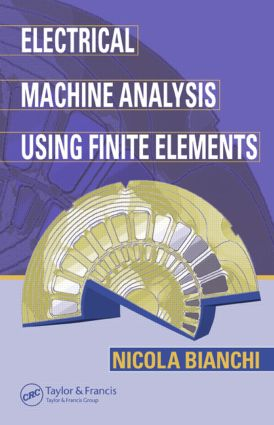# Electrical Machine Analysis Using Finite Elements

## 1st Edition

CRC Press

304 pages | 160 B/W Illus.

##### Purchasing Options:\$ = USD
Hardback: 9780849333996
pub: 2005-06-17
SAVE ~\$29.00
\$145.00
\$116.00
x
eBook (VitalSource) : 9781315219295
pub: 2017-12-19
from \$72.50

FREE Standard Shipping!

### Description

From the fan motor in your PC to precision control of aircraft, electrical machines of all sizes, varieties, and levels of complexity permeate our world. Some are very simple, while others require exacting and application-specific design. Electrical Machine Analysis Using Finite Elements provides the tools necessary for the analysis and design of any type of electrical machine by integrating mathematical/numerical techniques with analytical and design methodologies.

Building successively from simple to complex analyses, this book leads you step-by-step through the procedures and illustrates their implementation with examples of both traditional and innovative machines. Although the examples are of specific devices, they demonstrate how the procedures apply to any type of electrical machine, introducing a preliminary theory followed by various considerations for the unique circumstance. The author presents the mathematical background underlying the analysis, but emphasizes application of the techniques, common strategies, and obtained results. He also supplies codes for simple algorithms and reveals analytical methodologies that universally apply to any software program.

With step-by-step coverage of the fundamentals and common procedures, Electrical Machine Analysis Using Finite Elements offers a superior analytical framework that allows you to adapt to any electrical machine, to any software platform, and to any specific requirements that you may encounter.

OUTLINE OF ELECTROMAGNETIC FIELDS

Vector Analysis

Electromagnetic Fields

Fundamental Equations Summary

References

BASIC PRINCIPLES OF FINITE ELEMENT METHODS

Introduction

Field Problems with Boundary Conditions

Classical Method for the Field Problem Solution

The Classical Residual Method (Galerkin's Method)

The Classical Variational Method (Rayleigh-Ritz's Method)

The Finite Element Method

References

APPLICATIONS OF THE FINITE ELEMENT METHOD TO TWO-DIMENSIONAL FIELDS

Introduction

Linear Interpolation of the Function f

Application of the Variational Method

Simple Descriptions of Electromagnetic Fields

Appendix: Integration in Triangular Elements

THE ANALYSIS PROCEDURE USING THE FINITE ELEMENT METHOD

Introduction

Reduction of the Field Problem to a Two-Dimensional Problem

Boundary Conditions

Computation of the Solved Structure

CYLINDRICAL MAGNETIC DEVICES

Introduction

Analytical Study of the Magnetic Device

Finite Element Analysis

Example

References

THE SINGLE-PHASE TRANSFORMER

The Single-Phase Transformer

Equivalent Electric Circuit of the Transformer

Computation of the No-Load Inductances

Determination of the Leakage Inductances

Algorithm for the Construction of the Magnetizing Characteristic of the Transformer

References

SINGLE-PHASE VARIABLE REACTANCE

The Single-Phase Variable Reactance

Computation of the Reactance

Example

References

SYNCHRONOUS GENERATORS

Introduction

Computation of the No-Load Characteristic

Computation of the Direct-Axis Inductance

Computation of the Quadrature Axis Inductance

Self- and Mutual Inductances

Saturation Effect

Computation of Ld and Lq with Any Current

Computation of the Machine Characteristics

Example

Appendix: The Transformation abc-dq

References

SURFACE-MOUNTED PERMANENT MAGNET MOTORS

Introduction

Computation of the No-Load Characteristic

Computation of the Inductances

Computation of the Torque

Example

References

Further References on AC Drives

INTERIOR PERMANENT MAGNET AND RELUCTANCE SYNCHRONOUS MOTORS

Introduction

Characteristic Motor Parameters

Torque-Speed Characteristic

Algorithm for an Automatic Computation

Synchronous Reluctance Motors

References

SELF-STARTING SINGLE-PHASE SYNCHRONOUS MOTORS

Introduction

Definition of the Motor Model

Computation of the Electrical Parameters

Computation of the Torque

Analysis of the Dynamic Performance

Example

Two-Dimensional Linear Interpolation

Numerical Codes for the Motor Analysis

References

SWITCHED RELUCTANCE MOTORS

Introduction

Operating Principle

Field Problem Statement

Computation on the Solved Structure

Example

References

THREE-PHASE INDUCTION MOTORS

Introduction

Simulations of the Indirect Tests

Motor Analysis Using Simulations Under Load

References

APPENDIX

INDEX

### Subject Categories

##### BISAC Subject Codes/Headings:
TEC007000
TECHNOLOGY & ENGINEERING / Electrical
TEC008000
TECHNOLOGY & ENGINEERING / Electronics / General